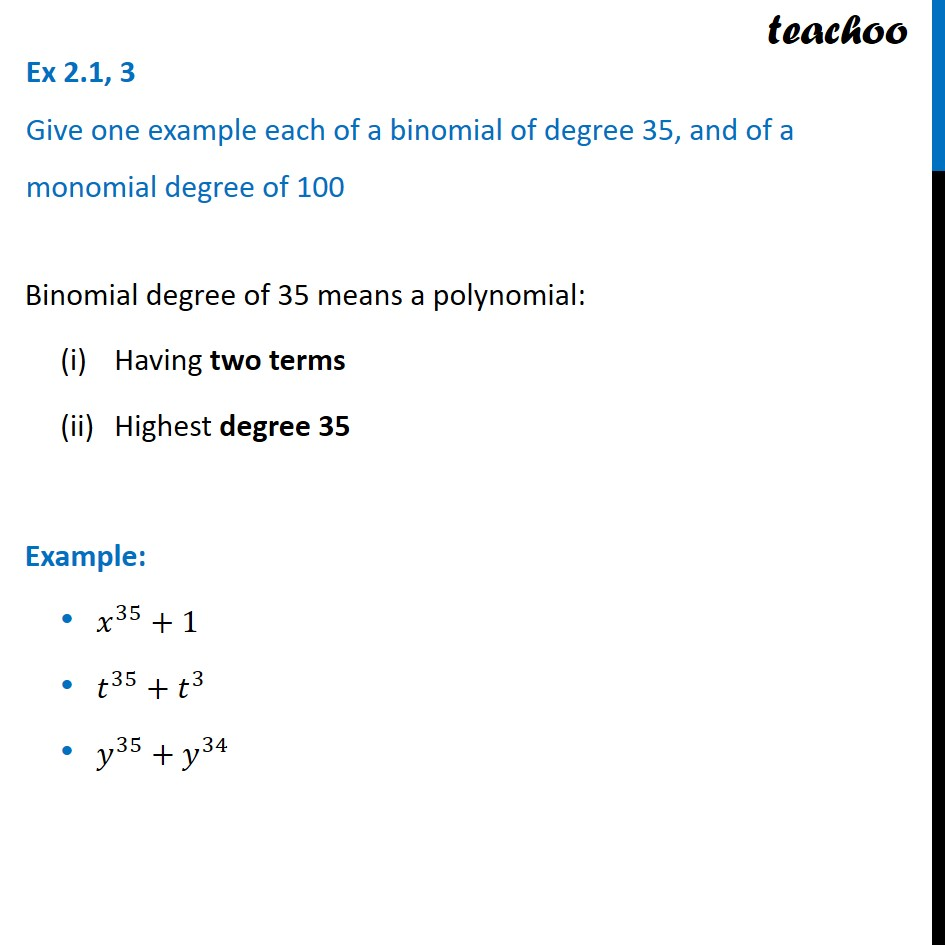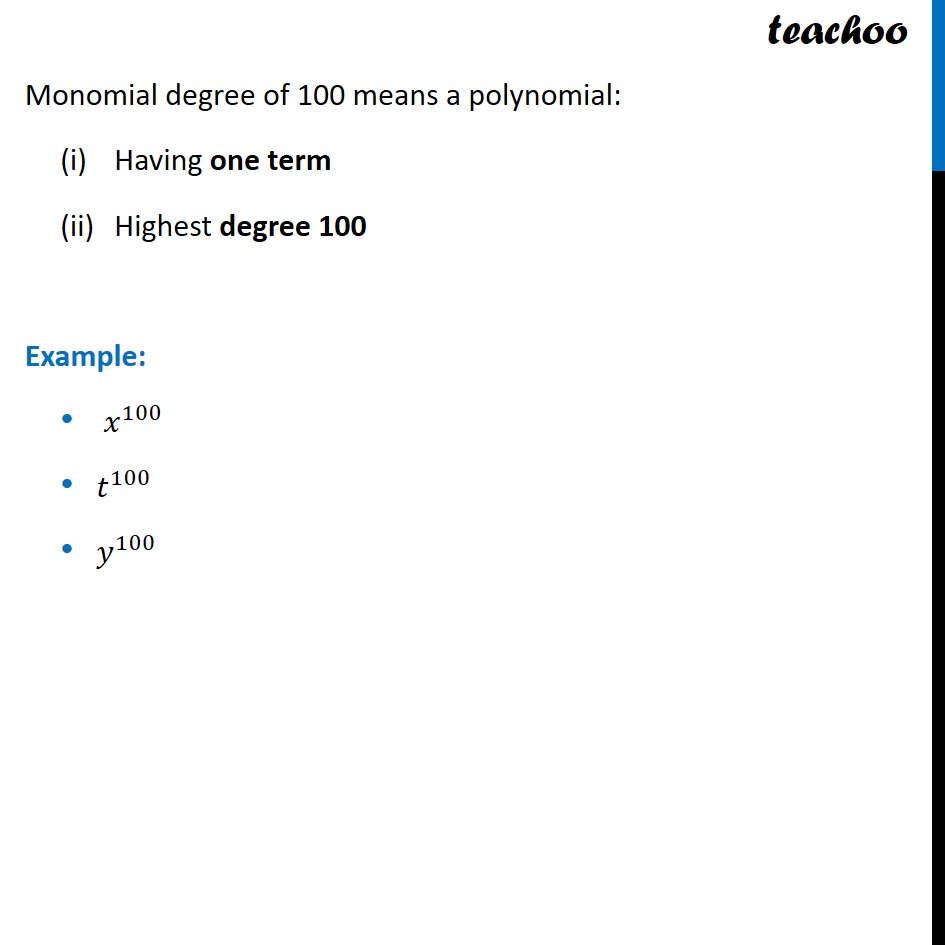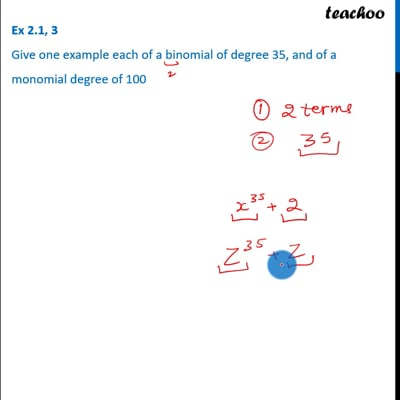Degree & Coefficient of a polynomial

Chapter 2 Class 9 Polynomials (Term 2)
Concept wiseThis video is only available for Teachoo black users

### Transcript

Ex 2.1, 3 Give one example each of a binomial of degree 35, and of a monomial degree of 100 Binomial degree of 35 means a polynomial: Having two terms Highest degree 35 Example: 𝑥^35+1 𝑡^35+𝑡^3 𝑦^35+𝑦^34 Monomial degree of 100 means a polynomial: Having one term Highest degree 100 Example: 𝑥^100 𝑡^100 𝑦^100